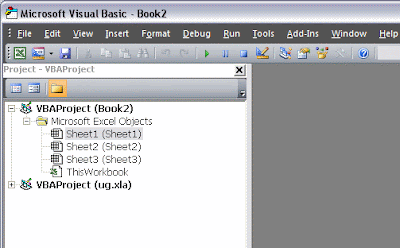Improve math skills of your kids - Learn step-by-step arithmetic from Math games

Math: Unknown - Step-by-step math calculation game for iOS.

Math: Unknown is much more than a math game. It is a step-by-step math calculation game which will teach users how to calculate in the correct order rather than just asking only the final calculated results.

The app consists of four basic arithmetic operations which are addition, subtraction, multiplication and division. In order to get started, users who are new to arithmetic can learn from animated calculation guides showing step-by-step procedures of solving each type of operation. It is also helpful for experienced users as a quick reference.

Generally, addition and subtraction may be difficult for users who just start learning math especially when questions require carrying or borrowing (also called regrouping). The app helps users to visualize the process of carrying and borrowing in the way it will be done on paper. Once users understand how these operations work, they are ready to learn multiplication and division.

For most students, division is considered as the most difficult arithmetic operation to solve. It is a common area of struggle since it requires prior knowledge of both multiplication and subtraction. To help users understand division, the app uses long division to teach all calculation procedures. Relevant multiplication table will be shown beside the question. Users will have to pick a number from the table which go into the dividend. Multiplication of selected number and divisor is automatically calculated, but the users have to do subtraction and drop down the next digit themselves. Learning whole calculation processes will make them master it in no time.

Math: Unknown is a helpful app for students who seriously want to improve arithmetic calculation skills.

Motion Simulation in Solid Edge using sketches together with Microsoft Excel VBA Programming Part III

In previous example, I showed how to control variables in Solid Edge sketch using direct link to excel spreadsheet. In this example, I am going to show how to use VBA (Visual Basic for Application) in excel to control the variables in Solid Edge sketch.

1) Create new file in Microsoft Excel

2) Press Alt+F11 to enter Microsoft Visual Basic windows as shown below3) Right click in Project Explorer at new file name (in this example, new excel file name = Book2) > Insert > Module4) To make Solid Edge type libraries available to the Visual Basic Object Browser, click References on the Project menu. On the References dialog box, select the Solid Edge libraries you want to access.All variable automation is accessed through the Variables collection and Variable objects. The Variables collection serves two purposes: it allows you to create and access variable objects, and it allows you to work with dimensions as variables.

Note: When debugging programs that interact with the Variable Table, it helps to have the Variable Table displayed while stepping through the program. The Variable Table shows the results as they are executed.

5) Write the following code into VBA code area as shown below,You can copy & paste the following code to your excel VBA.

' =================================================================
' Example of using VBA code to control variables in Solid Edge document
' By AkeBlogger
' http://mechanical-design-handbook.blogspot.com/
' =================================================================

Sub Simulate()
On Error Resume Next

'Declare the program variables.
Dim iAngle As Single
Dim objApp As Object
Dim objVariables As Object
Dim objVariable As Object

'------------------------------------------------------------------
' Checking for the active document
Set objApp = GetObject(, "SolidEdge.Application")
If Err Then
MsgBox "You must open Solid Edge before executing this module!!", vbOKOnly + vbExclamation, "Error"
Exit Sub
Else
'Connect to a running instance of Solid Edge.
Set objApp = GetObject(, "SolidEdge.Application")
'Access the Variables collection.
Set objVariables = objApp.ActiveDocument.Variables
End If

'SIMULATION STARTS!
For iAngle = 0 To 360 Step 2
' Changing Cam_angle from 0, 2, 4, ..., 360 deg.
Call objVariables.Edit("Cam_angle", iAngle)

Next iAngle

End Sub

6) Open Solid Edge file from previous example and delete formula in Variables Table as shown below, because in this example we will control the variable (dimension) using VBA code directly.7) We are now ready for simulation. Resize VBA Windows as shown below then click run to see the result.Watch the following video for the actual result!